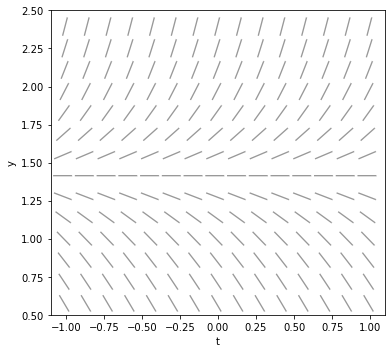# Numerical methods for ODEs¶

This notebook illustrates the numerical solution of Ordinary Differential Equations using Python. In particular, we'll see

• How to use SciPy's odeint command,
• How to visualize those solutions,
• How to convert the output of odeint to a computable function, and
• How to implement Euler's method.

Some of this will be immediately helpful for your homework and, hopefully, will be helpful throughout the class.

## Imports¶

Here are the libraries we'll be using:

In :
# A graphics library
import matplotlib.pyplot as plt

# Basic numerical tools
import numpy as np

# Scipy's numerical ODE solver
from scipy.integrate import odeint

# Convert data generated by odeint to a computable function
from scipy.interpolate import interp1d

# A numerical rootfinder
from scipy.optimize import brentq


## ODEINT¶

SciPy's odeint command is the most important tool we're learning here and we will return to it throughout the semester. Today, we'll apply it to just single, first order equations but we will learn later how to apply it to first order systems and higher order equations as well.

### A problem to explore¶

Here's an interesting growth equation:

$$y' = y\,e^{-y}.$$

As this is an autonomous equation, let's take a look at a plot of $f(y) = y\,e^{-y}$:

In :
# Define the function f
def f(y):
return y*np.exp(-y)

# Generate a range of 100 t values from 0 to 8
t = np.linspace(0,8,100)

# Apply f to get the corresponding y values
y = f(t)

# Plot y vs t
plt.plot(t,y);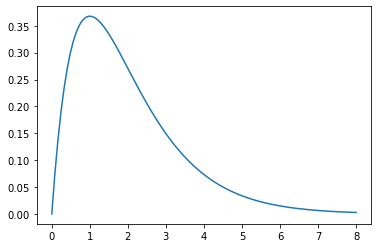I guess the growth rate is zero when the population is zero, increases as the population increases, but then tapers off as the population moves past 1. Note that the growth rate never hits zero (like the logistic equation) but it tapers off quite quickly.

#### An associated IVP¶

Now let's add the initial condition $y(0)=0.01$ so we can examine the plot of a specific solution; thus, we're studying

$$y' = y\,e^{-y}; \: y(0) = 0.01.$$

#### Using ODEINT¶

That IVP is exactly the kind of problem that odeint is built for. Let's solve the IVP and plot the solution straight away:

In :
# Redefine f as a function of both y and t
def f(y,t):
return y*np.exp(-y)

# Some t values that are appropriate to plot the solution
t = np.linspace(0,100,1000)

# Solve it!
y = odeint(f, 0.01, t).flatten()

# Plot it!!
plt.plot(t,y);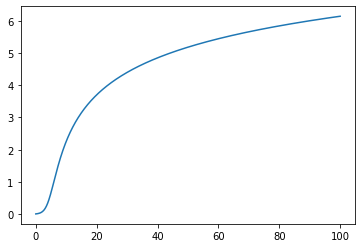This looks kinda like we might have expected. The population continues to grow but that growth rate slows down.

Note that the flatten at the end of odeint(f, 0.01, t).flatten() is not, strictly speaking, necessary to plot the solution. The point of flatten is that it "flattens" the output of odeint from a column vector to a 1D array. Let's look at the first few terms of the odeint result:

In :
odeint(f, 0.01, t)[:8]

Out:
array([[0.01      ],
[0.01104125],
[0.01218961],
[0.0134558 ],
[0.01485155],
[0.01638971],
[0.01808431],
[0.01995063]])

And here that is flattened:

In :
odeint(f,0.01,t)[:8].flatten()

Out:
array([0.01      , 0.01104125, 0.01218961, 0.0134558 , 0.01485155,
0.01638971, 0.01808431, 0.01995063])

The fact that odeint returns a vector has everything to do with the fact that odeint is really built to solve systems, rather than just single equations. For now, though, we'll consider the solution as a flat array, which is the form required by interp1D to interpolate those points to a numeric function. We can do that as follows:

In :
y_interp = interp1d(t,y)
y_interp

Out:
<scipy.interpolate.interpolate.interp1d at 0x11ecece00>

That rather mysterious looking output refers to a numerical routine that computes numerical estimates to values of the function. For example, here's the value of the solution at $t=20$:

In :
y_interp(20)

Out:
array(3.70195605)

Now both the graph and the computations indicate that the solution increases from $y(0) = 0.01$ to $y(20)\approx3.7$. We might ask the question - when, precisely, does the solution hit $y=3$? Put another way, for what value of $t_0$ is

$$y(t_0) = 3?$$

To answer this question, we use one of SciPy's numerical root finders, namely brentq. It's probably worth mentioning that you can get information on all the commands we're learning using the command? syntax. For example:

In :
brentq?


In particular, note that brentq uses a bracketing method so that it expects the endpoints of an interval where the function changes sign, as well as the function itself. Since our function doesn't change sign, we'll need to use a shifted version of our function to is equal to zero where are function is equal to three; that is we just shift down three units. It's pretty common to use so-called lambda functions for this purpose:

In :
t0 = brentq(lambda y: y_interp(y)-3, 0, 20)
t0

Out:
13.951879122196349

We can verify that this worked by simply plugging the point in:

In :
y_interp(t0)

Out:
array(3.)

We also might like a visual verification:

In :
plt.plot(t,y)
plt.plot([t0,t0],[0,6],'k--')
plt.plot([0,50],[3,3],'k--')
plt.plot(t0,3,'ro');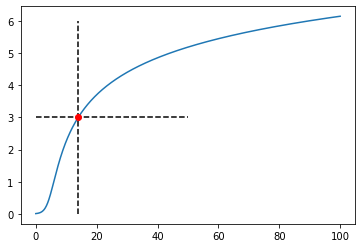The material to this point is everything you need to successfully complete our forum question.

## Euler's method¶

Euler's method, as we've learned, is the most basic method for solving an IVP - just follow the slope field. In quantitative terms, if we are trying to draw the graph of the solution of $y'=f(y,t)$ and we know that $(t_k,y_k)$ is on the graph, then we can generate a point $(t_{k+1},y_{k+1})$ (approximately) on the graph by

$$t_{k+1} = t_k + dt \: \text{ and } \: y_{k+1} = y_{k} + f(y_k,t_k)dt.$$

Note that second equation literally says that

$$y'(t_k) = f(y_k,t_k) \approx \frac{y_{k+1}-y_k}{dt},$$

i.e., the derivative is approximated by the difference quotient. So, this is pretty simple conceptually. It's also pretty easy to implement.

Let's try this for the equation above.

In :
# Define the function
def f(y,t):
return y*np.exp(-y)

# Define the initial conditions
t0 = 0
y0 = 0.01

# Define the stepsize
dt = 0.1

# Initialize the current tn and yn
tn = t0
yn = y0

# Initialize the list of ts and ys
ts = [t0]
ys = [y0]

# Perform the iteration
for i in range(100):
yn = yn + f(yn,tn)*dt # Next y
tn = tn + dt          # Next t
ys.append(yn)         # Append y
ts.append(tn)         # Append t


OK, let's look at the first bit of our solution:

In :
ys[:8]

Out:
[0.01,
0.010990049833749169,
0.012077042824688647,
0.013270249332157287,
0.014579780642723847,
0.016016655917475333,
0.01759287253035413,
0.019321479535164807]

Hmm... Let's plot it together with the odeint solution.

In :
plt.plot(ts,ys, 'r', linewidth=3)
plt.plot(t[:101],y[:101], 'g', linewidth=3);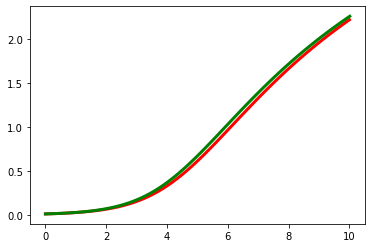Not bad! Let's look at the difference:

In :
plt.plot(ts,ys-y[:101].ravel());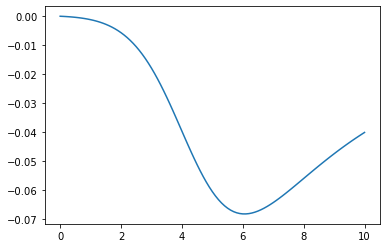Note that you can use this code to help on your most recent MyOpenMath assignment!

## Forwards and backwards through the slopefield¶

Adding a slopefield to our pictures takes a bit more work but is definitely worth it. Drawing a slopefield gets harder when your equation is non-autonomous. For example, let's take a look at:

$$y' = t+t^2-\frac{1}{10}y^3, \: \: y(0)=0.$$

We're also interested in dealing with an initial condition that's not at either endpoint of the domain. To do so we solve the problem twice - once forward and once backward. Here's how:

In :
# The right side of the ODE
def f(y,t): return t+t**2 - y**3/10

# A parameter specifying the size of the domain
r = 2

# Solve forward
ts_p = np.linspace(0,r,21)
sol_p = odeint(f,0,ts_p)

# Solve backward (note that ts_m goes from 0 down to -r)
ts_m = np.linspace(0,-r, 21)
sol_m = odeint(f,0,ts_m)

# Plot both parts of the solution
plt.plot(ts_m,sol_m, linewidth=2)
plt.plot(ts_p,sol_p, linewidth=2);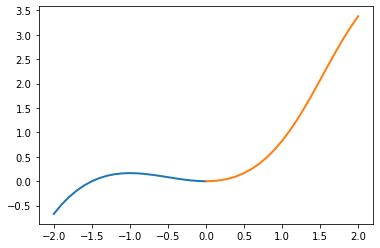Now, we'll draw the slope field using MatPlotLib's quiver command. Again, if you're curious about this, just execute plt.quiver?.

In :
T,Y = np.meshgrid( np.linspace(-r,r,10),np.linspace(-r,r,10) )
U = 1
V = f(Y,T)
N = np.sqrt(U**2+V**2)
U2, V2 = U/N, V/N
plt.quiver(T,Y,U2, V2,
alpha=0.4);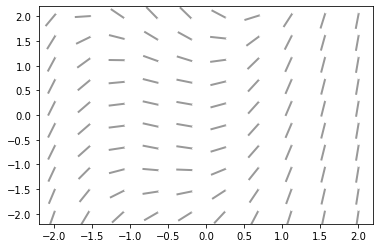Let's put those both together with some formatting info:

In :
plt.plot(ts_m,sol_m, linewidth=2)
plt.plot(ts_p,sol_p, linewidth=2)
plt.quiver(T,Y,U2, V2,
alpha=0.4);
ax=plt.gca()
ax.set_xlim(-r,r)
ax.set_ylim(-r,r)
ax.set_aspect(1)
ax.set_xlabel("t")
ax.set_ylabel("y")
fig = ax.get_figure()
fig.set_figheight(8);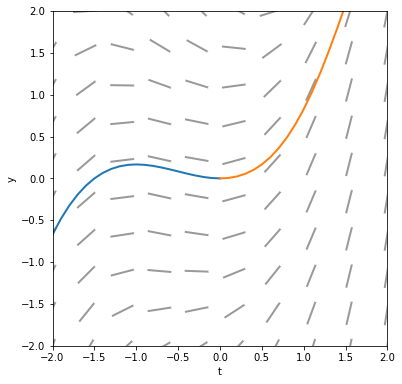Nice!

## Throwing off Euler's method¶

It's not too terribly hard to throw Euler's method off. Consider the following IVP.

\begin{align} y' &= 4\pi y^2 \\ y(0) &= -1 \end{align}

There is one semi-stable equilibrium solution, $y(t)=0$, as we can easily see from the slope field. Solutions below this equilibrium should increase up to the equilibrium. Let's apply Euler's method with a step size of $0.1$ and see what happens.

In :
# The right hand side of the ODE:
def f(y,t): return 4*np.pi*y**2

# Plot the slopefield
T,Y = np.meshgrid( np.linspace(-0.5,1.5,15),np.linspace(-1,1,15) )
V = f(Y,T)
N = np.sqrt(1**2+V**2)
U2, V2 = 1/N, V/N
plt.quiver(T,Y,U2, V2,
alpha=0.4)
ax=plt.gca()
ax.set_xlim(-0.5,1.5)
ax.set_ylim(-1,1)
ax.set_aspect(1)
ax.set_xlabel("t")
ax.set_ylabel("y")
fig = ax.get_figure()
fig.set_figheight(8)

# Euler's method:
t0 = 0
y0 = -1
tn = t0
yn = y0
dt = 0.1
ts = [t0]
ys = [y0]
for i in range(10):
yn = yn + f(yn,tn)*dt
tn = tn + dt
ys.append(yn)
ts.append(tn)
plt.plot(ts,ys)
ax = plt.gca()
ax.set_ylim(-1.1,1.1);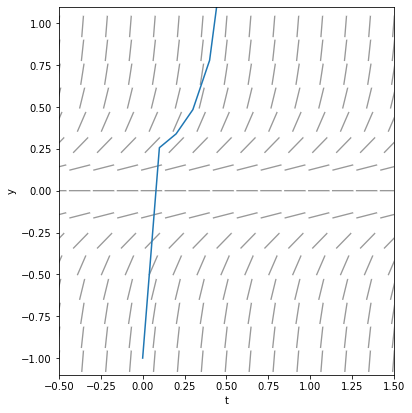Uh-oh - we jumped right over the equilibrium solution. Generally, the error associated with Euler's method decreases with decreasing stepsize. Still, this seems like something to be aware of.

## Throwing off odeint¶

Surely, odeint must be immune to our attempts to mess with it. Well, let's try to solve

\begin{align} y' &= y^2 - 2 \\ y(0) &= \sqrt{2}. \end{align}

Clearly the solution should be constant, since $y(t)=\sqrt{2}$ is an equilibrium solution.

In :
def f(y,t): return y**2 - 2
ts = np.linspace(0,10)
ys = odeint(f,np.sqrt(2),ts)
plt.plot(ts,ys);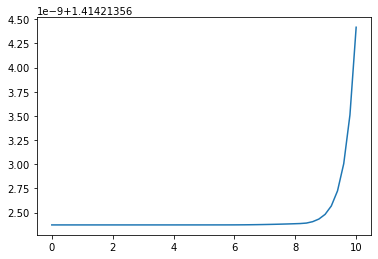Crazy! The deal is that, while $\sqrt{2}$ is an equilibrium, it is an unstable equilibrium. You can see this easily enough in the slope field.

In :
T,Y = np.meshgrid( np.linspace(-1,1,15), np.linspace(np.sqrt(2)-1,np.sqrt(2)+1,15))
V = f(Y,T)
N = np.sqrt(1**2+V**2)
U2, V2 = 1/N, V/N
plt.quiver(T,Y,U2, V2,